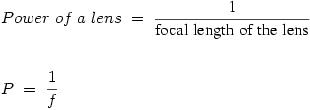Courses

# Test: Spherical Lens & its Power

## 10 Questions MCQ Test General Science(Prelims) by IRS Divey Sethi | Test: Spherical Lens & its Power

Description
This mock test of Test: Spherical Lens & its Power for UPSC helps you for every UPSC entrance exam. This contains 10 Multiple Choice Questions for UPSC Test: Spherical Lens & its Power (mcq) to study with solutions a complete question bank. The solved questions answers in this Test: Spherical Lens & its Power quiz give you a good mix of easy questions and tough questions. UPSC students definitely take this Test: Spherical Lens & its Power exercise for a better result in the exam. You can find other Test: Spherical Lens & its Power extra questions, long questions & short questions for UPSC on EduRev as well by searching above.
QUESTION: 1

### The combination of a convex lens of power +2D and a concave lens of power -2D, acts as a

Solution:

Net power =0
F=infinity
The emergent ray in the rectangular glass slab is parallel to the incident ray so the focal length of the glass slab is infinite.

QUESTION: 2

### A person having myopic eyes uses a concave lens of focal length 50 cm. What is the power of the lens ?

Solution:

So  focal length = - 50 cm = - 0.50 meter
power = - 1/0.50 = -2 Dioptre or - 2 D

QUESTION: 3

### The power of a lens of focal length 50 cm is

Solution:

Power of a lens is always reciprocal to the focal length. So,  a convex lens with 50 cm focal length has a power of :- 1/0.5
⇒  2D

QUESTION: 4

Power of a lens is

Solution:

Power of a Lens
The power of a lens is its ability to bend light – the greater the power the greater the refraction of light.

The power of a lens is also related to the focal length by the following formula:Power of a lens is measured in Dioptres (D).

Focal length is measured in metres (m).

QUESTION: 5

SI unit of power of lens is

Solution:
QUESTION: 6

The objective lens of microscope use

Solution:

The correct option is C.
The simplest compound microscope is constructed from two convex lenses. The objective lens is a convex lens of short focal length (i.e., high power) with typical magnification from 5× to 100×. The eyepiece, also referred to as the ocular, is a convex lens of longer focal length.

QUESTION: 7

A spherical mirror and a thin spherical lens have each a focal length of -12 cm. The mirror and the lens are likely to be:

Solution:

The focal length of concave mirror and lens is always negative.

QUESTION: 8

Which one of the following is the use of convex lens

Solution:
QUESTION: 9

Which of the following term is not associated with a lens ?

Solution:

Aperture of a lens is the length of the surface of lens exposed for incident light. Focal length is the distance between focus of the lens and lens optical center. There is no term like efficiency of a lens.

QUESTION: 10

Two thin lenses of power +3.5 D and -2.5 D are placed in contact. Find the focal length of the lens combination.​

Solution:

Power one of lens = P1 = +3.5D (Given)
Power two of lens = P2 = -2.5D (Given)
Power combination = P1 + P2

Thus,
Power of combination = +3.5 D +(-2.5 D )
= 3.5 D -2.5 D
= 1 D

Power = 1/focal length(m)
1 = 1/f
f = 1/1
f = 1 metre
Thus, the  f of combination = 1×100 = 100 cm

Therefore, if two thin lenses of power +3.5D and -2.5D are placed in contact then the power and focal length of the lens combination will be 100cm.